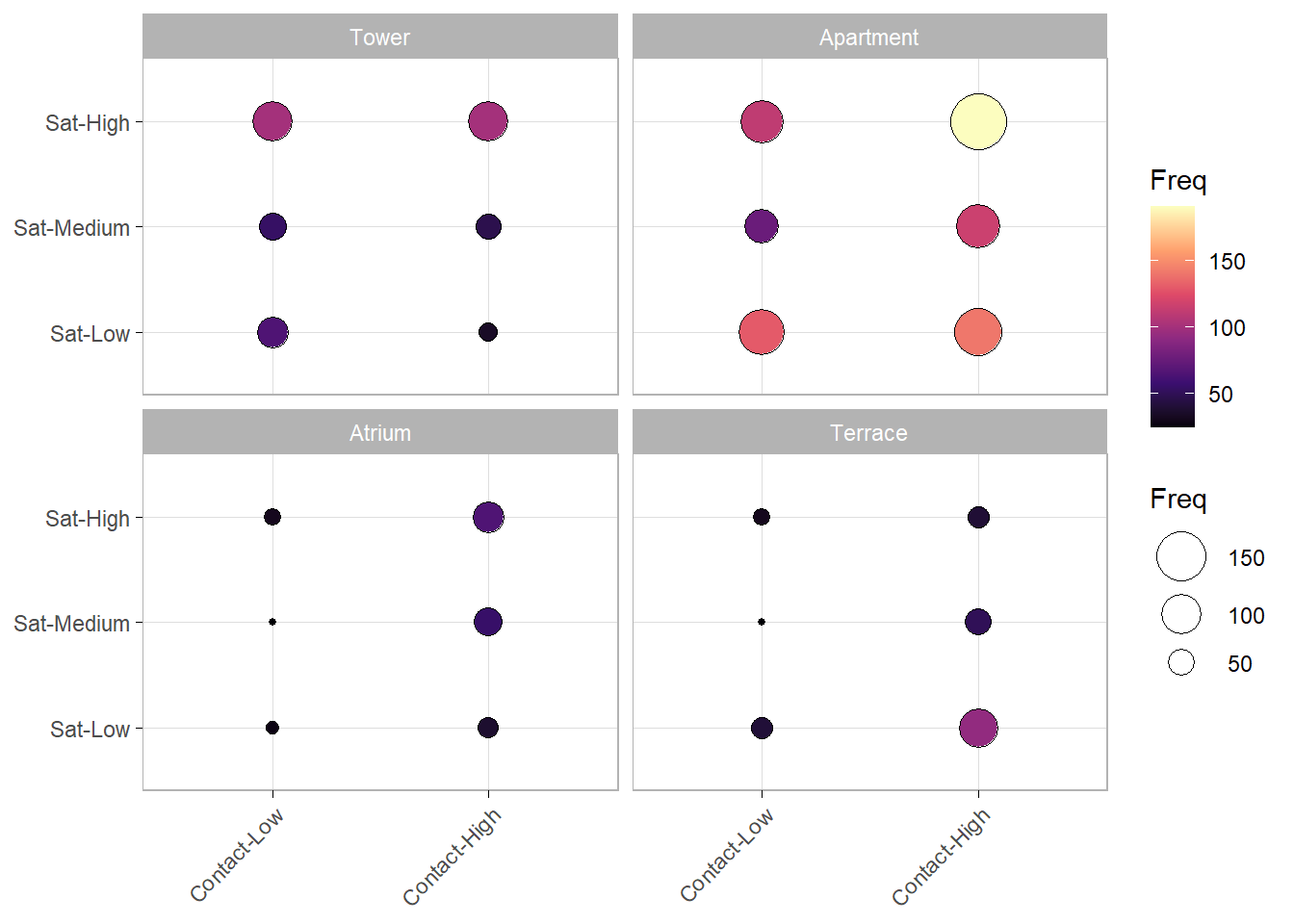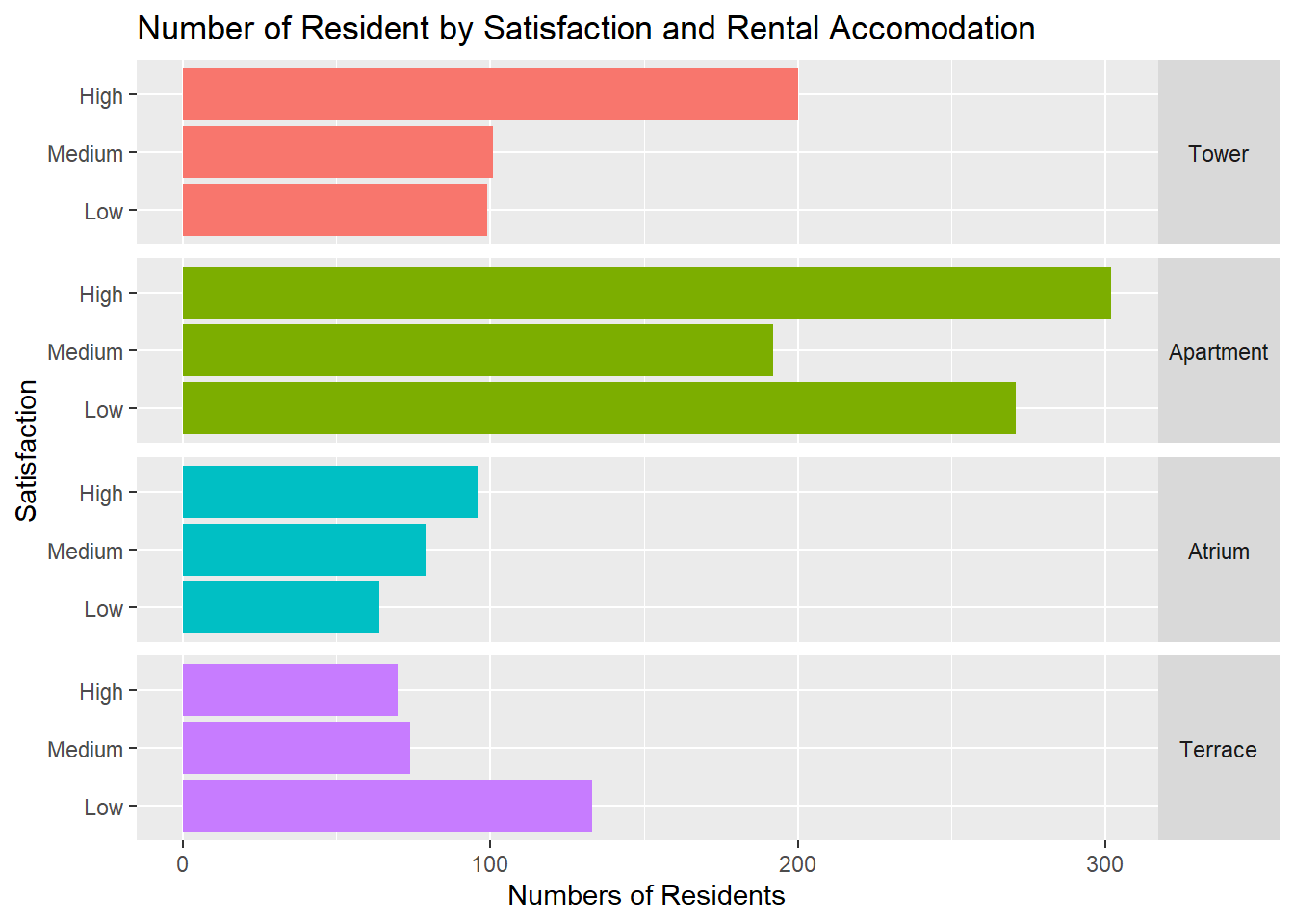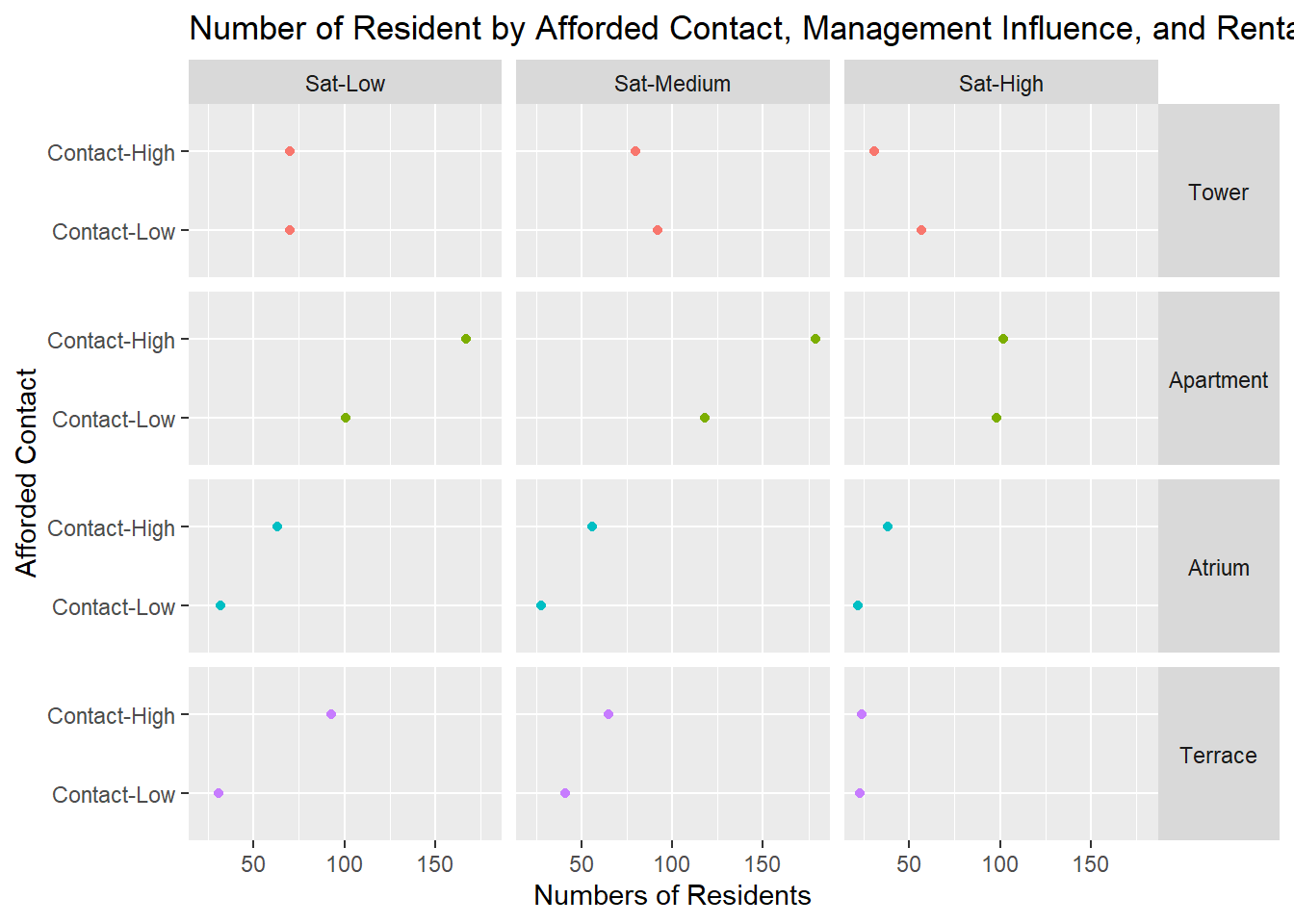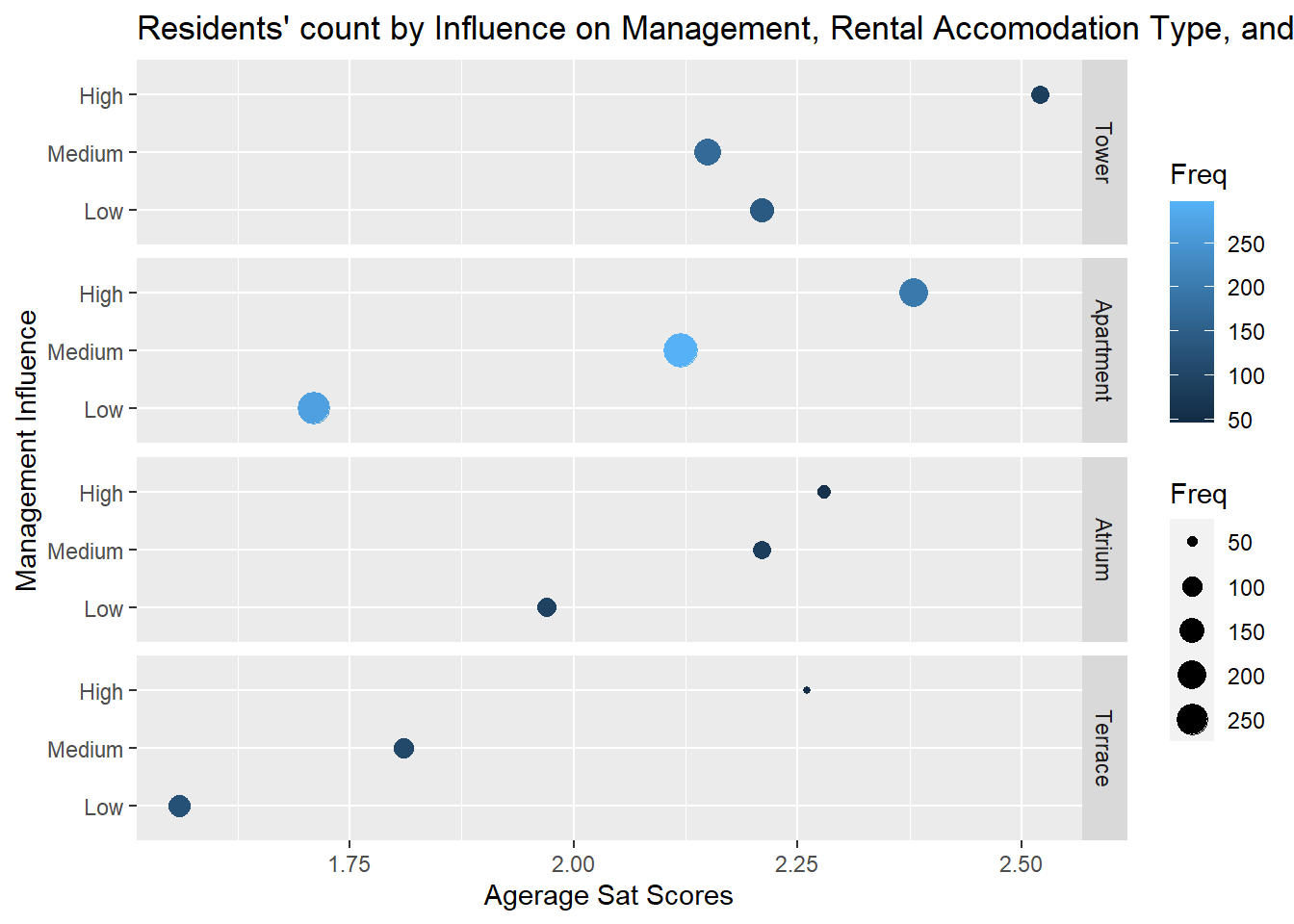## Objectives

• The objective of this assignment is to conduct an exploratory data analysis of a data set that you are not familiar with. In this week’s lecture, we discussed a number of visualization approaches in order to explore a data set with categorical variables. This assignment will apply those tools and techniques. An important distinction between class examples and applied data science work is iterative and repetitive nature of exploring a data set. It takes time to understand what the data is and what is interesting about the data (patterns).

• For this week, we will be exploring the Copenhagen Housing Conditions Survey:

• Sat - satisfaction of householders with their present housing circumstances
• Infl - perceived degree of influence householders have on the management of the property
• Type - type of rental accomodation
• Cont - contact residents are afforded with other residents
• Freq - Frequencies: the numbers of residents in each class
• Your task for this assignment is to use ggplot and the facet_grid and facet_wrap functions to explore the Copenhagen Housing Conditions Survey. Your objective is to develop 5 report quality visualizations (at least 4 of the visualizations should use the facet_wrap or facet_grid functions) and identify interesting patterns and trends within the data that may be indicative of large scale trends. For each visualization you need to write a few sentences about the trends and patterns that you discover from the visualization.

To submit this homework you will create the document in Rstudio, using the knitr package (button included in Rstudio) and then submit the document to your Rpubs account. Once uploaded you will submit the link to that document on Canvas. Please make sure that this link is hyperlinked and that I can see the visualization and the code required to create it.

## Look at the data

str(housing)
## 'data.frame':    72 obs. of  5 variables:
##  $Sat : Ord.factor w/ 3 levels "Low"<"Medium"<..: 1 2 3 1 2 3 1 2 3 1 ... ##$ Infl: Factor w/ 3 levels "Low","Medium",..: 1 1 1 2 2 2 3 3 3 1 ...
##  $Type: Factor w/ 4 levels "Tower","Apartment",..: 1 1 1 1 1 1 1 1 1 2 ... ##$ Cont: Factor w/ 2 levels "Low","High": 1 1 1 1 1 1 1 1 1 1 ...
levels(data2$Cont) = list('Contact-Low' = 'Low', 'Contact-High' = 'High') ggballoonplot(data2,x="Cont",y="Sat", size="Freq", facet.by="Type",fill="Freq",ggtheme=theme_light())+ scale_fill_viridis_c(option="A")## 3. Third plot # place code for vis here data3 = housing %>% group_by(Sat, Type) %>% summarise(Freq = sum(Freq)) ggplot(data3, aes(Freq, Sat)) + geom_bar(aes(fill=Type),stat='identity', position=position_dodge(0.9))+ facet_grid(Type ~ ., scales = 'fixed', space = 'fixed') + theme(strip.text.y = element_text(angle = 0), legend.position = 'none') + labs(y = 'Satisfaction', x = 'Numbers of Residents', title = 'Number of Resident by Satisfaction and Rental Accomodation')## 4. Fourth plot # place code for vis here data4 = housing %>% group_by(Type, Cont,Infl) %>% summarise(Freq = sum(Freq)) levels(data4$Infl) = list('Sat-Low' = 'Low', 'Sat-Medium' = 'Medium', 'Sat-High' = 'High')
levels(data4\$Cont) = list('Contact-Low' = 'Low', 'Contact-High' = 'High')

ggplot(data4, aes(Freq, Cont)) +
geom_point(aes(color=Type))+
facet_grid(Type ~ Infl, space="fixed", scales="fixed") +
theme(strip.text.y = element_text(angle = 0),
legend.position = 'none') +
labs(y = 'Afforded Contact',
x = 'Numbers of Residents',
title = 'Number of Resident by Afforded Contact, Management Influence, and Rental Accomodation')## 5. Fifth plot

# place code for vis here
data5 = housing %>%
mutate( stat_score=ifelse(Sat=="Low",1,ifelse(Sat=="High",3,2))* Freq
)%>%
group_by(Type,Infl)%>%
summarise(
stat_score=sum(stat_score),
Freq=sum(Freq)
)%>%
mutate(average=round(stat_score/Freq,2))

# Freq, average score, Type, Infl

ggplot(data5,aes(x=average, y=Infl))+
geom_point(aes(size=Freq,color=Freq))+
facet_grid(Type ~ .) +
labs(x="Agerage Sat Scores",
y="Management Influence",
title="Residents' count by Influence on Management, Rental Accomodation Type, and Average Satisfaction Scores")